# Fun with DAX – Minesweeper

The latest addition to my recent series of DAX based games is the classic Minesweeper game.  This is the game where you are presented with a 9 x 9 matrix of squares.  There are 10 hidden mines and you can either step on a square or place a flag where you think there might be a mine.  If you are lucky enough not to step on a square that contains a mine, you will get clues that help you identify where the mines are.

Click here if you would like to see the final publish to web version.

Once again, graphics and UI was provided by Mike Cairo over at PowerBI.Tips and make a huge difference to the look and feel of the game.  This is not just true of games like this.  If you want to make your business reports look more professional, it’s well worth heading over to PowerBI.Tips and checking out the fantastic pre-built layouts that you can download and try out with your data.I recognised early on with this game that the trickiest challenge would be deciding what squares to reveal.  I opted for the native Matrix visual to control the squares.  I forced the rows/columns to be 9 x 9 by creating a table with those numbers. The next thing was to create 81 tables to keep track of squares the player has either stepped on or flagged as a mine.   Each table represents a square on the matrix and carries a single column/ two-row table with a 1 or a 2.  A selection of 1 would denote a player has stepped on the square that table represents, while a 2 shows a flag has been placed.

Then I created 81 slicers over the top of the 81 tables that would be hidden.  Each slicer would be controlled by 2 bookmarks – meaning 162 bookmarks for flag/step control.  One bookmark for stepping on the square, while the other for flagging.

SVG images were generated for the squares for the various states.  These were all the same size, so once added to the matrix, I could begin to fit the invisible buttons to the board.  Care was taken to ensure the pixel layout was as close a fit as possible to the matrix loaded with images.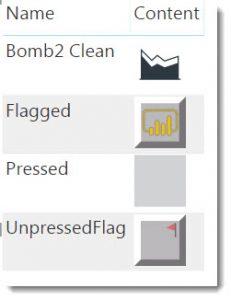There are two invisible buttons over each square on the board.  The first covers the whole square (approx 75 x 75 pixels) and has an action linked to the bookmark that sets the corresponding slicer to equal 1.  This records the player has “stepped” on the square.

A second smaller bookmark covers the top righthand corner of the button (40 x 40 pixels) and has an action linked to the bookmark that sets the corresponding slicer to equal 2.  This records the player has “flagged” the square as a mine.There is no concept of a left-click/right-click option in Power BI visuals, so decided this wasn’t a terrible way to give the player the two options needed for gameplay.

There are a lot of objects needed for a 9×9 matrix and currently no way to script.  This is why there is no option to chose a more advanced version of the game that includes more rows and columns.

The game logic is all contained in the [Super Measure] calculated measure.  This is the only measure that is added to the game matrix and will output the appropriate image depending on its position on the matrix taking into account the state of the 81 tables.  At the start of the game, none of the tables has a selection made so the initial output for every square is the “Unpressed” SVG.

One challenge I needed to solve was how to reveal a square as part of a region of blank squares.  In Minesweeper, if you click a square with a mine, it blows up.  If you click a square next to a mine, it reveals a number.  However, if you click a blank square, all blank squares and surrounding number squares are revealed.   So if were to click the top left square of the game and it happens to be a blank square then all connecting blank squares along with numbers should be revealed.This means the calculated measure needs a way to understand if “unpressed” squares on the board have a direct relationship with a blank square that has been pressed.  There can be multiple regions on any given game board and these can have quite irregular shapes.  I considered using nested iterators to try and work out on the fly but settled on a calculated column based approach to identify the regions.

A side note at this point is the 10 random locations of the mines are generated in Power Query.  This is done in Power Query rather than DAX so the 10 locations are set at the start and cannot change.  If this logic were in DAX the location of the mines would jump every time a filter is changed – which is not ideal.  The mines are stored in a table called ‘Mines’.

The co-ordinates used to identify each square starts with 1 for the top right square and then increments left to right and top to bottom.You may notice there are no squares for 10, 20, 30 etc.  I skipped these to make it easier for me to keep track of proximity values.  So for any given square I could apply the following filter adjustments to find its immediate neighbours in each direction.

-11 : above to the left
-10 : directly above
-9 : above to the right
-1 : left
+1 : right
+9 below to the left
+10 : directly below
+ 11: below to the right

In fact, I created a table with these numbers that I incorporate into the game logic to help keep the code tidy when needing to filter/identify nearby squares.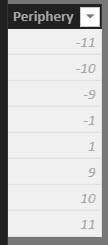The DAX for the calculated ‘Game logic ‘ table is as follows

```Game Logic =
VAR T =
GENERATESERIES(1,90),
"Y",INT(([Value]-1)/10),
"X",VAR x = MOD([Value],10) RETURN IF(x>0,x,10)
)
VAR T1 =
T ,
"Mine" ,
LOOKUPVALUE(
Mines[Column1],Mines[Column1],[Value]))
VAR T2 =
T1 ,
"Numbers" ,

COUNTROWS(GENERATE(p,FILTER(T1,EARLIER(''[Value])+[Periphery] = [Value] && [Mine] > 0)))
)
RETURN
filter(T2,[X]<>10)
```

This creates a base table with 5 columns to determine the row and column for each square, along with info showing if it is a Mine square, or carries a clue in the form of a number.

The badly named T1 variable adds the logic to show if the cell is a mine, while the T2 variable creates a filtered table on the fly using the [Periphery] logic to count the number of rows that happen to be a mine.From here, I use a series of columns, starting with a base set to help determine the regions of blank squares.  The trick is to make every square in the region have the same value.  I decided it might be easiest to take whatever the minimum coordinate is for the region and have that propagate out (like a virus!).

First I added a [Base Region] calculated column that only carries a value if the square is not a mine or clue square.

```Base Region =
IF(
ISBLANK('Game Logic'[Mine]) &&
ISBLANK('Game Logic'[Numbers]
),'Game Logic'[Value])```

When plotted on a matrix, you can see there are two distinct regions, but they have nothing in common at this point.

Now for the magic. The next column looks at every value in the same horizontal row and updates to be the minimum value for any value in that row.  This is smart enough to understand when unrelated regions share the same row.

```H1 =
VAR myX = 'Game Logic'[X]
VAR myY = 'Game Logic'[Y]
VAR myTable = FILTER('Game Logic','Game Logic'[Y] = myY)
VAR LowerBoundForMe =
VAR b =
MAXX(
FILTER(
myTable,
'Game Logic'[X] <= myX &&
ISBLANK('Game Logic'[Base Region])
),
'Game Logic'[Value]
)
return if(ISBLANK(b),0,b)

VAR UpperBoundForMe =
VAR b =
MINX(
FILTER(
myTable,
'Game Logic'[X] >= myX &&
ISBLANK('Game Logic'[Base Region])
),
'Game Logic'[Value]
)
RETURN IF(ISBLANK(b),99,b)
RETURN
MINX(
FILTER(
myTable,
'Game Logic'[Value] > LowerBoundForMe &&
'Game Logic'[Value] < UpperBoundForMe
),
'Game Logic'[Base Region]      --< Previous Column
)```

When this column is applied to the matrix we see the following.  Note how every row now carries the value that was the minimum value for that row.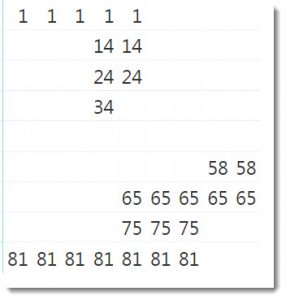Step 2 is to take the same calculated column approach but apply vertically.  This calculation is practicially identical to the above, only it uses the [Y] axis.

```V1 =
VAR myX = 'Game Logic'[X]
VAR myY = 'Game Logic'[Y]
VAR myTable = FILTER('Game Logic','Game Logic'[X] = myX)
VAR LowerBoundForMe =
VAR b =
MAXX(
FILTER(
myTable,
'Game Logic'[Y] <= myY &&
ISBLANK('Game Logic'[Base Region])
),
'Game Logic'[Y]
)
RETURN IF(ISBLANK(b),-1,b)

VAR UpperBoundForMe =
VAR b =
MINX(
FILTER(
myTable,
'Game Logic'[Y] >= myY &&
ISBLANK('Game Logic'[Base Region])
),
'Game Logic'[Y]
)
RETURN IF(ISBLANK(b),99,b)
RETURN
MINX(
FILTER(
myTable,
'Game Logic'[Y] > LowerBoundForMe &&
'Game Logic'[Y] < UpperBoundForMe
),
'Game Logic'[H1]
)```

Note the top region is showing all 1’s now so is complete.  While the bottom region has a mix.  Eventually, the bottom region will show all 58’s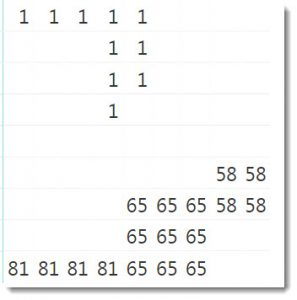I repeat the same technique applying horizontally then vertically.  Each column builds on the results of the last columns.

So horizontal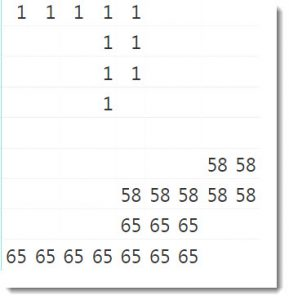Then verticalfinally horizontalI can keep this going as many times as I need but for a 9×9 grid, I suspect only 3 passes of pairs are needed.

Once the numbers have been established, I rank the regions based on their lowest number and convert the ranking (1,2 or 3) to an alpha character (A, B or C).  I do this to make it easier for me to distinqish a region square from a numberic clue square.

```Region =
VAR TableToRank = FILTER(ALL('Game Logic'[V3]),NOT ISBLANK('Game Logic'[V3]))
VAR RegionChar = UNICHAR(RANKX(TableToRank,'Game Logic'[V3],,ASC) + 64)
RETURN
IF(NOT ISBLANK('Game Logic'[V3]),RegionChar)```

The final column combines mines, clues and region squares into a single column that when added to the matrix looks like:The last piece of the puzzle is to create a measure to control what is displayed on the game matrix.

```Super Measure =
VAR myY =
MIN ( 'Game Logic'[Y] )
VAR myX =
MIN ( 'Game Logic'[X] )
VAR myValue =
MIN ( 'Game Logic'[Value] )
VAR myRegion =
MIN ( 'Game Logic'[Region] )
VAR mySteppedValue =
MIN ( 'Game Logic'[Step Value] )
VAR FlaggedSquares =  SELECTCOLUMNS (
FILTER (
UNION (
{ IF ( SELECTEDVALUE ( 'Table R1C1'[Value] ) = 2, 1 ) },
{ IF ( SELECTEDVALUE ( 'Table R1C2'[Value] ) = 2, 2 ) },
{ IF ( SELECTEDVALUE ( 'Table R1C3'[Value] ) = 2, 3 ) },
{ IF ( SELECTEDVALUE ( 'Table R1C4'[Value] ) = 2, 4 ) },
{ IF ( SELECTEDVALUE ( 'Table R1C5'[Value] ) = 2, 5 ) },
{ IF ( SELECTEDVALUE ( 'Table R1C6'[Value] ) = 2, 6 ) },
{ IF ( SELECTEDVALUE ( 'Table R1C7'[Value] ) = 2, 7 ) },
{ IF ( SELECTEDVALUE ( 'Table R1C8'[Value] ) = 2, 8 ) },
{ IF ( SELECTEDVALUE ( 'Table R1C9'[Value] ) = 2, 9 ) },
----------------------------------------------
<... CODE REMOVED FOR BREVITY - PLEASE SEE PBIX FILE FOR FULL CODE ...>
----------------------------------------------
{
IF ( SELECTEDVALUE ( 'Table R9C1'[Value] ) = 2, 81 )
},
{ IF ( SELECTEDVALUE ( 'Table R9C2'[Value] ) = 2, 82 ) },
{ IF ( SELECTEDVALUE ( 'Table R9C3'[Value] ) = 2, 83 ) },
{ IF ( SELECTEDVALUE ( 'Table R9C4'[Value] ) = 2, 84 ) },
{ IF ( SELECTEDVALUE ( 'Table R9C5'[Value] ) = 2, 85 ) },
{ IF ( SELECTEDVALUE ( 'Table R9C6'[Value] ) = 2, 86 ) },
{ IF ( SELECTEDVALUE ( 'Table R9C7'[Value] ) = 2, 87 ) },
{ IF ( SELECTEDVALUE ( 'Table R9C8'[Value] ) = 2, 88 ) },
{ IF ( SELECTEDVALUE ( 'Table R9C9'[Value] ) = 2, 89 ) }
),
[Value] > 0
),
"Stepped Square", [Value]
)
VAR SteppedSquares =
SELECTCOLUMNS (
FILTER (
UNION (
{ IF ( SELECTEDVALUE ( 'Table R1C1'[Value] ) = 1, 1 ) },
{ IF ( SELECTEDVALUE ( 'Table R1C2'[Value] ) = 1, 2 ) },
{ IF ( SELECTEDVALUE ( 'Table R1C3'[Value] ) = 1, 3 ) },
{ IF ( SELECTEDVALUE ( 'Table R1C4'[Value] ) = 1, 4 ) },
{ IF ( SELECTEDVALUE ( 'Table R1C5'[Value] ) = 1, 5 ) },
{ IF ( SELECTEDVALUE ( 'Table R1C6'[Value] ) = 1, 6 ) },
{ IF ( SELECTEDVALUE ( 'Table R1C7'[Value] ) = 1, 7 ) },
{ IF ( SELECTEDVALUE ( 'Table R1C8'[Value] ) = 1, 8 ) },
{ IF ( SELECTEDVALUE ( 'Table R1C9'[Value] ) = 1, 9 ) },
----------------------------------------------
<... CODE REMOVED FOR BREVITY ...>
----------------------------------------------
{
IF ( SELECTEDVALUE ( 'Table R9C1'[Value] ) = 1, 81 )
},
{ IF ( SELECTEDVALUE ( 'Table R9C2'[Value] ) = 1, 82 ) },
{ IF ( SELECTEDVALUE ( 'Table R9C3'[Value] ) = 1, 83 ) },
{ IF ( SELECTEDVALUE ( 'Table R9C4'[Value] ) = 1, 84 ) },
{ IF ( SELECTEDVALUE ( 'Table R9C5'[Value] ) = 1, 85 ) },
{ IF ( SELECTEDVALUE ( 'Table R9C6'[Value] ) = 1, 86 ) },
{ IF ( SELECTEDVALUE ( 'Table R9C7'[Value] ) = 1, 87 ) },
{ IF ( SELECTEDVALUE ( 'Table R9C8'[Value] ) = 1, 88 ) },
{ IF ( SELECTEDVALUE ( 'Table R9C9'[Value] ) = 1, 89 ) }
),
[Value] > 0
),
"Stepped Square", [Value]
)
VAR RegionsSelected =
DISTINCT (
SELECTCOLUMNS (
GENERATE (
SteppedSquares,
FILTER (
ALL ( 'Game Logic' ),
'Game Logic'[Value] = [Stepped Square]
&& 'Game Logic'[Region] <> ""
)
),
"DDD", [Region]
)
)
VAR RegionSteppedSquares =
GENERATE (
RegionsSelected,
FILTER ( ALL ( 'Game Logic' ), 'Game Logic'[Region] = [DDD] )
)
RETURN
SWITCH (
TRUE (),
-- Test 1 -- Detects if a mine has been stepped on
-- Show all mines
COUNTROWS (
GENERATE (
SteppedSquares,
FILTER (
ALL ( 'Game Logic' ),
'Game Logic'[Value] = [Stepped Square]
-- && 'Game Logic'[Region] = "X"
&& 'Game Logic'[Mine] > 0
)
)
) > 0 && COUNTROWS(FILTER('Game Logic','Game Logic'[Mine]=myValue)) > 0  ,
-- THEN --
[SVG Image Bomb],

--- TEST 2 ----
COUNTROWS (
GENERATE (
SteppedSquares,
FILTER (
ALL ( 'Game Logic' ),
'Game Logic'[Value] = [Stepped Square]
&& 'Game Logic'[Region] = myRegion
&& myRegion <> ""
)
)
)
> 0, -- then --
[SVG Image Pressed],
-- IF --
COUNTROWS (
FILTER ( FlaggedSquares, [Stepped Square] = myValue )
)
> 0,
[SVG Image Flagged],
-- IF -- This shows number
COUNTROWS (
FILTER ( SteppedSquares, [Stepped Square] = myValue )
)
> 0, -- THEN--
"data:image/svg+xml;utf8,<svg version='1.0' xmlns='http://www.w3.org/2000/svg' viewBox='0 0 80 80'>"
& "<text x='27' y='58' fill='" &
SWITCH(
TRUE(),
mySteppedValue="1","#198DDF",
mySteppedValue="2","#6E901D",
mySteppedValue="3","#E02872",
mySteppedValue="4","#2D59AA",
"#B62020"
) & "' style='font: bold 50px sans-serif;'>"
& mySteppedValue
& "</text>"
& "</svg>",
--mySteppedValue,
--- TEST 2 ----
-- IF -- This shows entire region
--- TEST 2 ----
-- IF -- Neighbour of Region (using P table to get periphery
COUNTROWS (
FILTER ( GENERATE ( RegionSteppedSquares, p ), [Value] = myValue + [Periphery] )
)
> 0,         "data:image/svg+xml;utf8,<svg version='1.0' xmlns='http://www.w3.org/2000/svg' viewBox='0 0 80 80'>"
& "<text x='27' y='58' fill='" &
SWITCH(
TRUE(),
mySteppedValue="1","#198DDF",
mySteppedValue="2","#6E901D",
mySteppedValue="3","#E02872",
mySteppedValue="4","#2D59AA",
"#B62020"
) & "' style='font: bold 50px sans-serif;'>"
& mySteppedValue
& "</text>"
& "</svg>",
--mySteppedValue,
-- ELSE --
[SVG Image Unpressed]
)```

This particular block of code probably deserves a blog in its own right.  The idea is to establish what SVG image the measure needs to output for the square.  There is a SWITCH statement near the end that applies some tests such as :

• Am I a mine square and have I been stepped on
• Am I a mine square and has another mine been stepped on
• Am I a clue square? If I am, choose and appropriate colour for my number
• Am I a blank square that has been stepped on
• Am I a blank square that is in the same region as a blank square that has been stepped on
• Am I a clue square that borders a region that has had a square stepped on

So variables prior to the final SWITCH statement are designed to make it easier to apply the above tests.

The last objects are measures that show progress on a panel on the left-hand side. These are managed by a [Flags Placed] and [Result] calculated measure.

All in all, it looks pretty good.  The grid seems to have some alignment issues that surface when the first move has been played but then goes away again once a second move has been made.  I’m not sure if this is to do with the measure drawing SVG images to the matrix.

I hope you like the game and find something interesting in the DAX and/or the approach.  Feel free to reach out on Twitter, or comment here if you would like clarification or more detail on any aspect of the game.

Phil is Microsoft Data Platform MVP and an experienced database and business intelligence (BI) professional with a deep knowledge of the Microsoft B.I. stack along with extensive knowledge of data warehouse (DW) methodologies and enterprise data modelling. He has 25+ years experience in this field and an active member of Power BI community.

## 6 thoughts on “Fun with DAX – Minesweeper”

•Patrick says:

Incredible stuff Phil!

•Teck Yong Tan says: